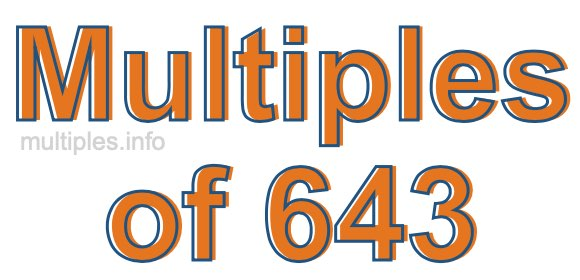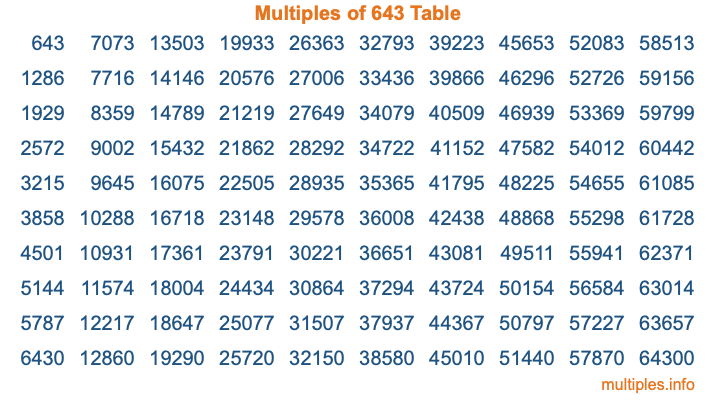Multiples of 643Welcome to the Multiples of 643 page. Here we will first teach you everything you will ever need to know about the multiples of 643, and then give you a study guide summary of everything we taught you to make sure you remember it all. Use this page to look up facts and learn information about the multiples of 643. This page will make you a multiples of six hundred forty-three expert!

Definition of Multiples of 643
Multiples of 643 are all the numbers that when divided by 643 equal an integer. Each of the multiples of 643 are called a multiple. A multiple of 643 is created by multiplying 643 by an integer.

Therefore, to create a list of multiples of 643, you start with 1 multiplied by 643, then 2 multiplied by 643, then 3 multiplied by 643, and so on for as long as you want. Thus, the list of the first five multiples of 643 is 643, 1286, 1929, 2572, and 3215. To see a larger list of multiples of 643, see the printable image of Multiples of 643 further down on this page. We also have a category where you can choose any nth multiple of 643.

Multiples of 643 Checker
The Multiples of 643 Checker below checks to see if any number of your choice is a multiple of 643. In other words, it checks to see if there is any number (integer) that when multiplied by 643 will equal your number. To do that, we divide your number by 643. If the the quotient is an integer, then your number is a multiple of 643.

Is  a multiple of 643?

Least Common Multiple of 643 and ...
A Least Common Multiple (LCM) is the lowest multiple that two or more numbers have in common. This is also called the smallest common multiple or lowest common multiple and is useful to know when you are adding our subtracting fractions. Enter one or more numbers below (643 is already entered) to find the LCM.

Check out our LCM Calculator if you need more details about the Least Common Multiple or if you need the LCM for different numbers for adding and subtraction fractions.

nth Multiple of 643
As we stated above, 643 is the first multiple of 643, 1286 is the second multiple of 643, 1929 is the third multiple of 643, and so on. Enter a number below to find the nth multiple of 643.

th multiple of 643

Multiples of 643 vs Factors of 643
643 is a multiple of 643 and a factor of 643, but that is where the similarities end. All postive multiples of 643 are 643 or greater than 643. All positive factors of 643 are 643 or less than 643.

Below is the beginning list of multiples of 643 and the factors of 643 so you can compare:

Multiples of 643: 643, 1286, 1929, 2572, 3215, etc.

Factors of 643: 1, 643

As you can see, the multiples of 643 are all the numbers that you can divide by 643 to get a whole number. The factors of 643, on the other hand, are all the whole numbers that you can multiply by another whole number to get 643.

It's also interesting to note that if a number (x) is a factor of 643, then 643 will also be a multiple of that number (x).

Multiples of 643 vs Divisors of 643
The divisors of 643 are all the integers that 643 can be divided by evenly. Below is a list of the divisors of 643.

Divisors of 643: 1, 643

The interesting thing to note here is that if you take any multiple of 643 and divide it by a divisor of 643, you will see that the quotient is an integer.

Multiples of 643 Table
Below is an image of the first 100 multiples of 643 in a table. The table is in chronological order, column by column. The first column has the first ten multiples of 643, the second column has the next ten multiples of 643, and so on.The Multiples of 643 Table is also referred to as the 643 Times Table or Times Table of 643. You are welcome to print out our table for your studies.

Negative Multiples of 643
Although not often discussed or needed in math, it is worth mentioning that you can make a list of negative multiples of 643 by multiplying 643 by -1, then by -2, then by -3, and so on, to get the following list of negative multiples of 643:

-643, -1286, -1929, -2572, -3215, etc.

Multiples of 643 Summary
Below is a summary of important Multiples of 643 facts that we have discussed on this page. To retain the knowledge on this page, we recommend that you read through the summary and explain to yourself or a study partner why they hold true.

There are an infinite number of multiples of 643.

A multiple of 643 divided by 643 will equal a whole number.

643 divided by a factor of 643 equals a divisor of 643.

The nth multiple of 643 is n times 643.

The largest factor of 643 is equal to the first positive multiple of 643.

643 is a multiple of every factor of 643.

643 is a multiple of 643.

A multiple of 643 divided by a divisor of 643 equals an integer.

643 divided by a divisor of 643 equals a factor of 643.

Any integer times 643 will equal a multiple of 643.

Multiples of a Number
Here you can get the multiples of another number, all with the same attention to detail as we did for multiples of 643 on this page.

Multiples of
Multiples of 644
Did you find our page about multiples of six hundred forty-three educational? Do you want more knowledge? Check out the multiples of the next number on our list!